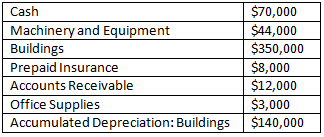# Problem: The chart lists accounts in no particular order. What are total current assets? a. $90,000 b.$93,000 c. $137,000 d.$347,000

###### Problem Details

The chart lists accounts in no particular order. What are total current assets?

a. $90,000 b.$93,000

c. $137,000 d.$347,000